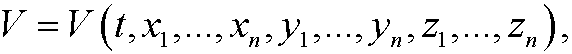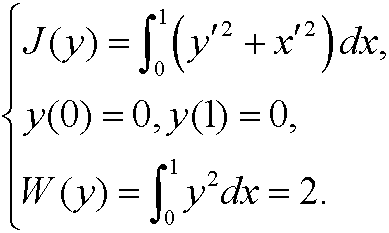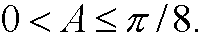# 10. Exercises. vii

7.1) Consider the functionalFind the Hamiltonian H(t,y,p) and state the canonical equations for the problem.

7.2) Derive Hamilton’s equations for the functionalrepresenting the motion of  a pendulum in the plane.

7.3) Consider a system of n particles where mi is the mass of the i:th particle and (xi,yi,zi) its position in space. The kinetic energy of the system isAssume that the system has potential energyso that the force acting on the i:th particle has the componentsin the x-y- and z-directions. Show that Hamilton’s principle imply the equationsNewton’s equations for a system of n particles.

7.4) The American post office prescribes that the sum of the length a and the circumference p=2b+2c of a cross section of a box may not exceed 100 inches.Find the proportions of the allowed box with maximal volume.

7.5) Compute the shortest distance d from a point on the ellipse x2+4y2=4to the line x+y=4.7.6) Find the extremals to the isoperimetric problem7.7) Determine the equation for the shortest curve in the first quadrant that passes through the points (0,0) and (1,0) and that together with the x-axis encloses a give area A, where7.8) Find the extremals to the isoperimetric problemunder the constraint